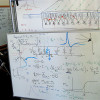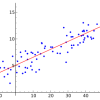Use the offer code 20offearlybird to get 20% off.## Clever Application Of A Predictive Model

What if you could use a predictive model to find new combinations of attributes that do not exist in the data but could be valuable. In Chapter 10 of Applied Predictive Modeling, Kuhn and Johnson provide a case study that does just this. It’s a fascinating and creative example of how to use a predictive […]## Improve Model Accuracy with Data Pre-Processing

Data preparation can make or break the predictive ability of your model. In Chapter 3 of their book Applied Predictive Modeling, Kuhn and Johnson introduce the process of data preparation. They refer to it as the addition, deletion or transformation of training set data. In this post you will discover the data pre-process steps that […]## Model Prediction Accuracy Versus Interpretation in Machine Learning

In their book Applied Predictive Modeling, Kuhn and Johnson comment early on the trade-off of model prediction accuracy versus model interpretation. For a given problem, it is critical to have a clear idea of the which is a priority, accuracy or explainability so that this trade-off can be made explicitly rather than implicitly. In this […]## Non-Linear Regression in R with Decision Trees

In this post, you will discover 8 recipes for non-linear regression with decision trees in R. Each example in this post uses the longley dataset provided in the datasets package that comes with R. The longley dataset describes 7 economic variables observed from 1947 to 1962 used to predict the number of people employed yearly. Let’s get started. Classification […]## Non-Linear Regression in R

In this post you will discover 4 recipes for non-linear regression in R. There are many advanced methods you can use for non-linear regression, and these recipes are but a sample of the methods you could use. Let’s get started. Each example in this post uses the longley dataset provided in the datasets package that comes with R. The […]## Penalized Regression in R

In this post you will discover 3 recipes for penalized regression for the R platform. You can copy and paste the recipes in this post to make a jump-start on your own problem or to learn and practice with linear regression in R. Let’s get started. Each example in this post uses the longley dataset provided in the datasets […]## Linear Regression in R

In this post you will discover 4 recipes for linear regression for the R platform. You can copy and paste the recipes in this post to make a jump-start on your own problem or to learn and practice with linear regression in R. Let’s get started. Each example in this post uses the longley dataset […]## Applied Machine Learning Lessons from A Case Study of Passenger Survival Prediction

A valuable exercise when learning and practicing machine learning is to study how others apply methods and solve problems. It’s valuable because you can learn about new processes, software, graphs, and algorithms. But it is new ways of thinking about the process of solving problems with machine learning that is the most valuable part of […]## Java Machine Learning

Are you a Java programmer and looking to get started or practice machine learning? Writing programs that make use of machine learning is the best way to learn machine learning. You can write the algorithms yourself from scratch, but you can make a lot more progress if you leverage an existing open source library. In […]## How to Tune Algorithm Parameters with Scikit-Learn

Machine learning models are parameterized so that their behavior can be tuned for a given problem. Models can have many parameters and finding the best combination of parameters can be treated as a search problem. In this post, you will discover how to tune the parameters of machine learning algorithms in Python using the scikit-learn […]